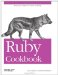# Taking Logarithms

Problem

You want to take the logarithm of a number, possibly a huge one.

Solution

Math.log calculates the natural log of a number: that is, the log base e.

```	Math.log(1) # => 0.0
Math.log(Math::E) # => 1.0
Math.log(10) # => 2.30258509299405
Math::E ** Math.log(25) # => 25.0
```

Math.log10 calculates the log base 10 of a number:

```	Math.log10(1) # => 0.0
Math.log10(10) # => 1.0
Math.log10(10.1) # => 1.00432137378264
Math.log10(1000) # => 3.0
10 ** Math.log10(25) # => 25.0
```

To calculate a logarithm in some other base, use the fact that, for any bases b1 and b2, logb1(x) = logb2(x) / logb2(k).

```	module Math
def Math.logb(num, base)
log(num) / log(base)
end
end
```

Discussion

A logarithm function inverts an exponentiation function. The log base k of x,or logk(x), is the number that gives x when raised to the k power. That is, Math. log10(1000)==3.0 because 10 cubed is 1000.Math.log(Math::E)==1 because e to the first power is e.

The logarithm functions for all numeric bases are related (you can get from one base to another by dividing by a constant factor), but they're used for different purposes.

Scientific applications often use the natural log: this is the fastest log implementation in Ruby. The log base 10 is often used to visualize datasets that span many orders of magnitude, such as the pH scale for acidity and the Richter scale for earthquake intensity. Analyses of algorithms often use the log base 2, or binary logarithm.

If you intend to do a lot of algorithms in a base that Ruby doesn't support natively, you can speed up the calculation by precalculating the dividend:

```	dividend = Math.log(2)
(1..6).collect { |x| Math.log(x) / dividend }
# => [0.0, 1.0, 1.58496250072116, 2.0, 2.32192809488736, 2.58496250072116]
```

The logarithm functions in Math will only accept integers or floating-point numbers, not BigDecimal or Bignum objects. This is inconvenient since logarithms are often used to make extremely large numbers managable. The BigMath module has a function to take the natural logarithm of a BigDecimal number, but it's very slow.

Here's a fast drop-in replacement for BigMath::log that exploits the logarithmic identity log(x*y)==log(x) + log(y). It decomposes a BigDecimal into three much smaller numbers, and operates on those numbers. This avoids the cases that give BigMath::log such poor performance.

```	require 'bigdecimal'
require 'bigdecimal/math'
require 'bigdecimal/util'

module BigMath
alias :log_slow :log
def log(x, prec)
if x <= 0 || prec <= 0
raise ArgumentError, "Zero or negative argument for log"
end
return x if x.infinite? || x.nan?
sign, fraction, power, exponent = x.split
fraction = BigDecimal(".#{fraction}")
power = power.to_s.to_d
log_slow(fraction, prec) + (log_slow(power, prec) * exponent)
end
end
```

Like BigMath::log, this implementation returns a BigMath accurate to at least prec digits, but containing some additional digits which might not be accurate. To avoid giving the impression that the result is more accurate than it is, you can round the number to prec digits with BigDecimal#round.

```	include BigMath

number = BigDecimal("1234.5678")
Math.log(number) # => 7.11847622829779

prec = 50

BigMath.log_slow(number, prec).round(prec).to_s("F")
# => "7.11847622829778629250879253638708184134073214145175"

BigMath.log(number, prec).round(prec).to_s("F")
# => "7.11847622829778629250879253638708184134073214145175"
BigMath.log(number ** 1000, prec).round(prec).to_s("F")
# => "7118.47622829778629250879253638708184134073214145175161"
```

As before, calculate a log other than the natural log by dividing by BigMath.log(base) or BigMath.log_slow(base).

```	huge_number = BigDecimal("1000") ** 1000
base = BigDecimal("10")
BigMath.log(huge_number, 100) / BigMath.log(base, 100)).to_f
# => 3000.0
```

How does it work? The internal representation of a BigDecimal is as a number in scientific notation: fraction*10**power. Because log(x*y)=log(x) + log(y), the log of such a number is log(fraction) + log(10**power).

10**power is just 10 multiplied by itself power times (that is, 10*10*10*…*10). Again, log(x*y)=log(x) + log(y), so log(10*10*10*…*10)=log(10)+log(10) + log(10)+…+log(10),or log(10)*power. This means we can take the logarithm of a huge BigDecimal by taking the logarithm of its (very small) fractional portion and the logarithm of 10.

• Mathematicians used to spend years constructing tables of logarithms for scientific and engineering applications; so if you find yourself doing a boring job, be glad you don't have to do that (see http://en.wikipedia.org/wiki/Logarithm#Tables_of_logarithms)Ruby Cookbook (Cookbooks (OReilly))
ISBN: 0596523696
EAN: 2147483647
Year: N/A
Pages: 399